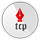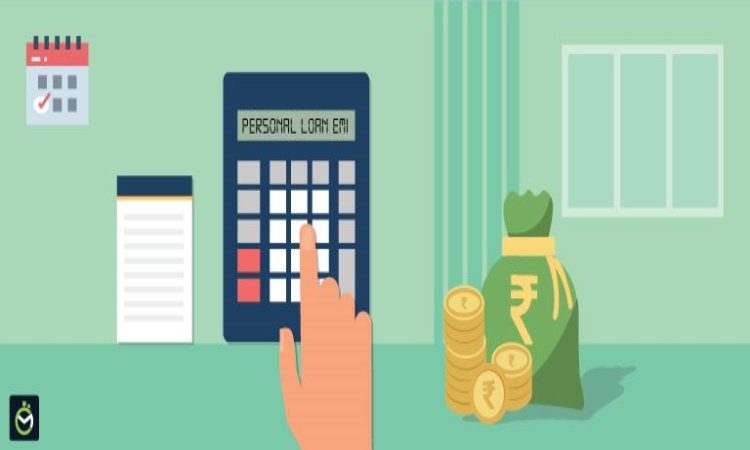﻿ Best Ways to Avail Personal Loan EMI Options Without Visiting Branch | Covaipost
09 Jun 2023, Edition - 2887, Friday

### Trending Now

• SC Collegium recommends names of 4 district judges for appointment as Madras HC judges
• Himachal Pradesh has all rights to impose cess on usage of water: Dy CM Mukesh Agnihotri
• JD(S) leader Kumaraswamy to meet Mamata Banerjee on Friday

# Best Ways to Avail Personal Loan EMI Options Without Visiting BranchCovai Post Network

•An urgent cash need may arise at any time in any form, be it a medical emergency, urgent home renovation, last-minute wedding expenses, spontaneous vacation, or study abroad. When such situations occur, people seek Personal Loans. These unsecured funding options give immediate access to cash while ensuring a hassle-free application process. However, knowing how to calculate EMI for Personal Loan is essential before applying. It will help in planning the finances appropriately and selecting the best loan offer in the market.

Here, we have covered three common methods of calculating Personal Loan EMIs without physically visiting the loan provider’s branch office.

Factors Affecting Loan EMIs

Whichever method an aspiring borrower uses to calculate the EMIs, they will need to provide three crucial details to make it happen. These include the following:

1. Principal Loan Amount:

Principal is the amount a borrower borrows as a loan. The lower the loan amount, the lower the EMIs charges. The loan amount directly impacts the payable EMIs.

2. Rate of Interest:

The rate at which the loan company approves or offers the loan. It is directly proportional to the loan EMIs. The higher the interest rate, the bigger the EMIs will be.

3. Loan Tenure:

The loan tenure is the period within which the borrower has to repay the loan. The tenure is inversely proportional to EMIs. A shorter term increases the EMI amount while a longer tenure decreases it, making the EMIs affordable for the borrower. However, it increases the total loan cost due to the increased number of EMIs in the tenure.

EMI Calculation Methods Without Visiting Branch

1. Calculating EMIs Manually Using a Mathematical Formula

The traditional method of calculating EMIs is manual, using a mathematical formula which is:

EMI = P x R x (1+R)^N / [(1+R)^N-1]

Here, P = Principal Amount, R = Rate of Interest, N = Number of months in the loan term
For instance, if an individual uses a Personal Loan eligibility calculator and borrows a loan of ₹ 2 Lakh at a 12% interest rate for a tenure of 24 months, the monthly EMI using the mathematical formula would be:
P = ₹ 2,000,00
N = 24 months
R = 0.01

EMI: 2,00,000 * 0.01 * (1 + 0.01)24 / ((1 + 0.01)24 – 1) = INR 10,333

The borrower will pay an EMI of ₹ 10,333 each month for two years or twenty-four months. Overall, the total loan cost would be ₹ 2,47,992, of which ₹ 48,000 (approx) will be the total interest cost charged on loan.

Although the manual method to calculate EMI for Personal Loan does not require a branch visit, it is cumbersome, time-consuming, and error-prone. If the user wants to adjust the loan tenure, they need to do all these calculations again to get the new result. Sometimes, users do not have enough mathematical skills to use the mathematical formula appropriately. Even a single mistake can change the outcome and affect the loan decision. Therefore, most people prefer automated ways to calculate EMIs and get faster and easier results with accuracy.

2. Calculating EMIs Automatically Using an Online EMI Calculator

The Personal Loan EMI calculator is an online tool that helps do the calculations without brain-wrestling. The calculator uses the mathematical formula in the background to calculate the EMIs and displays the results on the screen instantly. The user must visit the NBFC’s website or download the app, find the online Personal Loan EMI calculator, and enter the required details, including the loan amount, interest rate, and loan tenure.
Calculating EMIs using an EMI calculator is much better than doing it manually because of the following reasons:

 Gives a Better Understanding of the Loan Terms:

First-time borrowers may find it challenging to do all the complicated calculations. An EMI calculator makes it simple by letting aspiring borrowers easily understand a loan’s features and aspects.

 Saves Time:

Calculating loan EMIs using an online calculator will help save time significantly. Aspiring borrowers do not need to visit the lender’s office anymore. They can decide on the loan amount they want to borrow with a Personal Loan eligibility calculator and find their monthly obligations by entering a few values in the tool.

 Gives an Accurate Estimate of the EMI Amount:

Getting an accurate estimate of the EMI amount helps borrowers plan their finances accordingly. However, they must ensure that their DTI ratio is below 30-40% to maximise their chances of approval.

 Error-Free:

An automatic EMI calculator eliminates any chance of an error or miscalculation, giving users an accurate estimate each time they use the tool.

Aspiring borrowers can easily find an EMI calculator on an NBFC’s website or app and use it any number of times, free of charge.

3. Calculating EMIs Using PMT Method On Excel

The formula to calculate EMI for Personal Loan using Excel helps calculate the PMT (Present Value of Payments), which is equivalent to EMIs. The formula to use is:
=PMT (RATE, NPER, PV, FV, TYPE)
Other than PMT, all other elements in the formula are variables, and the user needs to assign them values according to their loan particulars. They must enter them carefully to get accurate results. Here is a breakdown of these variables:

 RATE: The applicable loan interest rate divided by 12

 NPER: The number of monthly EMI payments in the tenure

 PV: The principal amount or present value the user intends to borrow

 FV: The balance remainder or future value after the last payment

 TYPE: When the payment is due, ranging from 0 to 1